• 策略迭代：二维状态网格实现
2019-06-17 22:03:24
#参考：https://www.cnblogs.com/devilmaycry812839668/p/10314049.html
#encoding:UTF-8
#!/usr/bin/env python3

import random

#状态
states=[0,1,2,3,4,5]

#动作
actions=["a", "b"]

# 奖励的折扣因子
gama=0.9

""" 状态值  v_value
v_value={
"1":0,
"2":0
}"""
v_value={}
for state in states:
v_value[state]=0

# 动作值 ("1", "a"):0
q_value={}

#状态转移
def p_state_reward(state, action):
# 输入当前状态，及行为
# return 跳转概率，下一状态, 奖励
if state==0:
if action=="a":
return (0, 0, 0)
else:
return (0, 2, 0)
if state==1:
if action=="a":
return (1/2, 0, 1)
else:
return (1/2, 2, 0)
if state==2:
if action=="a":
return (1/2, 1, 0)
else:
return (1/2, 3, 0)
if state==3:
if action=="a":
return (1/2, 2, 0)
else:
return (1/2, 4, 0)
if state==4:
if action=="a":
return (1/2, 3, 0)
else:
return (1/2, 5, 5)
if state==5:
if action=="a":
return (0, 5, 0)
else:
return (0, 5, 0)

# q_value 初始值
"""q_value={
("1", "a"):(1.0/3*()),
("1", "b"):0,
("2", "a"):0,
("2", "b"):0
}"""
def q_value_fun():
q_value.clear()
for state in states:
for action in actions:
psr=p_state_reward(state, action)
temp=0
temp+=psr*(psr+gama*v_value[psr])
#for t in p_state_reward(state, action):总报错
#temp+=t*(t+gama*v_value[t])
q_value[(state, action)]=temp
#q_value初始化
q_value_fun()

#策略 pi 初始化   "1":{"a":0.5, "b":0.5}

pi={}
for state in states:
temp={}
for action in actions:
temp[action]=1.0/len(actions)
pi[state]=temp

#print(v_value)
#print(pi)
#print(q_value)

#策略评估 得出 v_value 值
def policy_evalue():
global v_value
v_value_new = {}

def v_update():
nonlocal v_value_new
v_value_new = {}
for state in states:
temp = 0
for action, p in pi[state].items():
temp += p * q_value[(state, action)]
v_value_new[state] = temp
# print("v_value:        "+str(v_value))
# print("v_value_new:    "+str(v_value_new))

#迭代停止条件
def stop_judge():
flag=True
for state, value in v_value.items():
if abs(v_value_new[state]-value)>0.0001:
flag=False
return flag

# 计算 v_value_new
v_update()

while stop_judge()!=True:
# 更新 v_value
v_value=v_value_new
# 更新 q_value
q_value_fun()
# 再次迭代 计算v_value_new
v_update()

#策略改进 max
def policy_improve():
flag=True
for state in states:
#L=[]
#for action in actions:
#    L.append((q_value[state, action], action))
#action=max(L)[-1]
action=max((q_value[state, action], action) for action in actions)[-1]

for k in pi[state]:
if k==action:
if pi[state][k]!=1.0:
pi[state][k]=1.0
flag=False
else:
pi[state][k]=0.0
return flag

if __name__=="__main__":
"""
policy_evalue()
print("*"*30)
print(v_value)
print("*"*30)
print(q_value)
print("*"*30)
print(pi)
"""
policy_evalue()
flag = policy_improve()
i = 1
while flag != True:
i += 1
policy_evalue()
flag = policy_improve()

#print("*" * 30 + "\n")
print("总共运行次数:" + str(i) + "\n")
print("状态值为：")
print(v_value)
print("")
print("状态行为值为：")
print(q_value)
print("策略为：")
print(pi)


更多相关内容
• 策略迭代算法和值函数迭代算法 文章目录1. 回顾与引言2. 思路介绍3. 策略评估算法3. 策略优化算法4. 策略迭代算法和值函数迭代算法5. 代码实现6. 强化学习与最优控制 1. 回顾与引言 上一章中介绍了马尔科夫决策过程...

策略迭代算法和值函数迭代算法

## 1. 回顾与引言

大家如果不了解马尔科夫决策过程可以先阅读这篇文章：https://blog.csdn.net/qq_33302004/article/details/115027798
上一篇文章中介绍了马尔科夫决策过程（MDP），也介绍了状态值函数和行为-状态值函数的计算方法。由此我们已经完成了对强化学习问题的建模过程，我们知道强化学习就是寻找一个最优策略 π \pi ，保证一个已知的MDP ( S , A , P , r , γ ) (S, A, P, r, \gamma) 的累计回报期望最大，也就是：
π = arg max ⁡ π ∫ R ( τ ) p π ( τ ) d τ \pi = \argmax_\pi \int {R(\tau)p_\pi(\tau)} d\tau
我们把已知状态转移概率 P P 的问题有模型问题，把未知 P P 的问题叫做无模型问题，由此最优化MDP的方法可分为基于模型的动态规划方法和基于无模型的强化学习方法，如下图所示：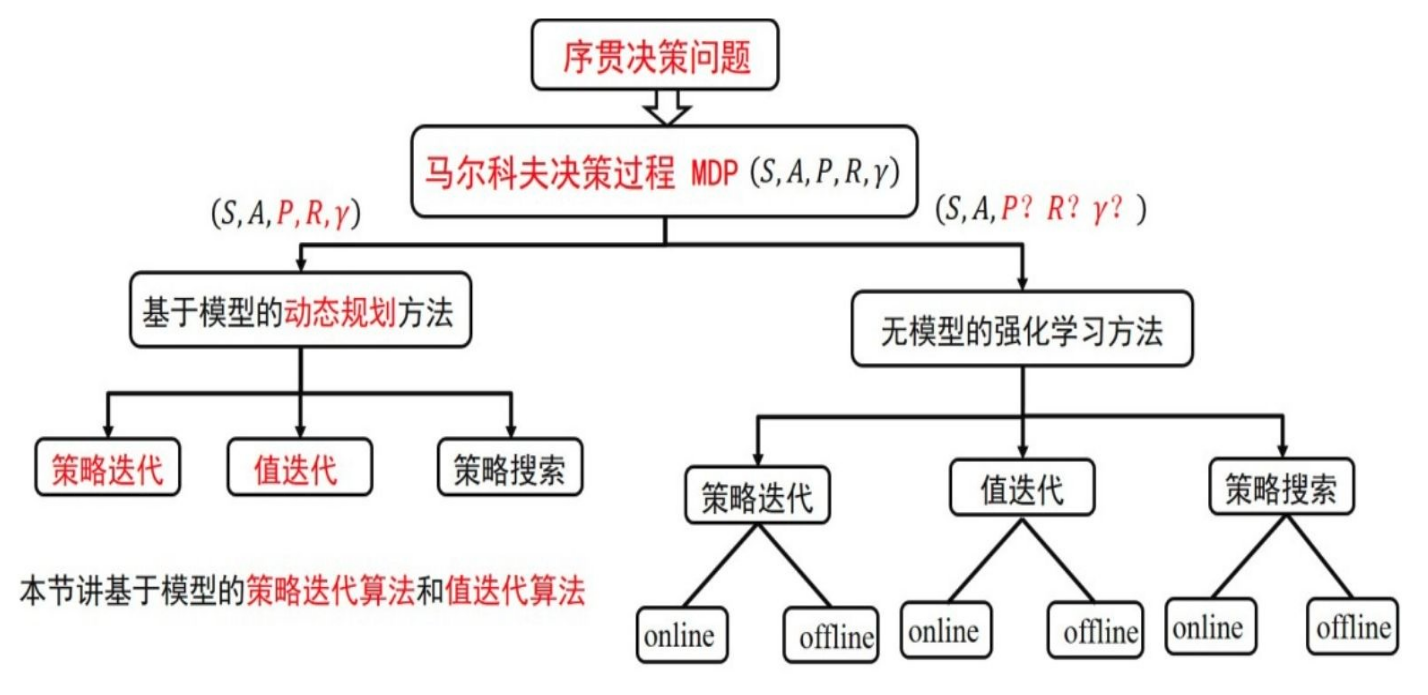由图中可知，这两种方法都包括策略迭代算法、值函数迭代算法、策略搜索算法。本文将介绍基于模型的策略迭代算法值函数迭代算法

## 2. 思路介绍

先不考虑策略迭代或者值函数迭代的概念，来回顾一下我们要解决的问题。在序贯决策问题中，我们知道全部的状态S、可以采用的全部动作A，还知道在状态S下采用动作A会转移到什么状态S‘（P），以及对应的反馈R和损失因子 γ \gamma 。我们现在我们需要考虑两个问题：

1. 如何产生一个策略 π \pi ，也就是： a = π ( s ) a = \pi(s) => 策略优化
2. 如何评价一个策略 π \pi 。 => 策略评估

我们手上有两个武器，状态值函数和行为-状态值函数：
ν π ( s ) = ∑ a ∈ A π ( a ∣ s ) ( R s a + γ ∑ s ′ ∈ S P s s ′ a ν π ( s ′ ) ) \nu_\pi(s) = \sum_{a\in A} \pi(a|s) \left( R_s^a + \gamma\sum_{s' \in S}P_{ss'}^a\nu_\pi(s') \right)
q π ( s , a ) = R s a + γ ∑ s ′ ∈ S P s s ′ a ν π ( s ′ ) q_\pi(s,a) = R_s^a + \gamma\sum_{s' \in S}P_{ss'}^a\nu_\pi(s')
通过状态值函数，我们可以评估策略 π \pi 当前的状态价值分布是如何的，通过行为-状态值函数，我们可以知道在当前状态下采用哪种动作更好，从而优化策略 π \pi

## 3. 策略评估算法

策略评估算法就是在已知一个策略 π \pi 的情况下，如何计算出每个状态的值函数。解决方案是采用“高斯-赛尔德迭代算法”求解状态值函数的迭代公式：
ν π ( s ) = ∑ a ∈ A π ( a ∣ s ) ( R s a + γ ∑ s ′ ∈ S P s s ′ a ν π ( s ′ ) ) \nu_\pi(s) = \sum_{a\in A} \pi(a|s) \left( R_s^a + \gamma\sum_{s' \in S}P_{ss'}^a\nu_\pi(s') \right)
在上面这个式子中出了状态值函数外，所有变量都是已知的，所以本质上就是一个解方程的过程。不理解“高斯-赛尔德迭代算法”的话，可以简单理解为： x = f ( x ′ ) x=f(x') ，本次的 x x 可以用上一次计算的 x ′ x' 求得，并且通过无限迭代最终会收敛，收敛处的 x x 值就是方程组的解。

策略评估算法的伪代码如下：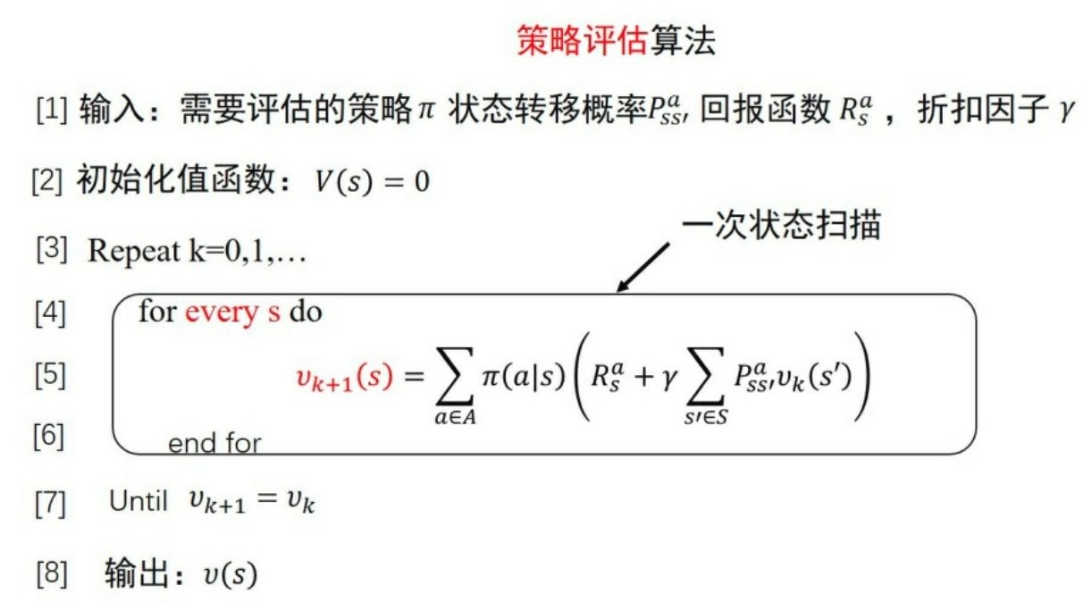我们来举个例子，如下图所示为网格世界，其状态空间为S={1,2,…,14}，动作空间为A={东,南,⻄,北}，回报函数为r≡-1，需要评估的策略为均匀随机策略，也就是东南西北选择的概率都为0.25。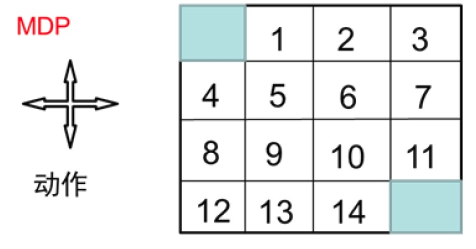迭代0次、1次、2次、3次后每个状态的值函数的值如下图所示：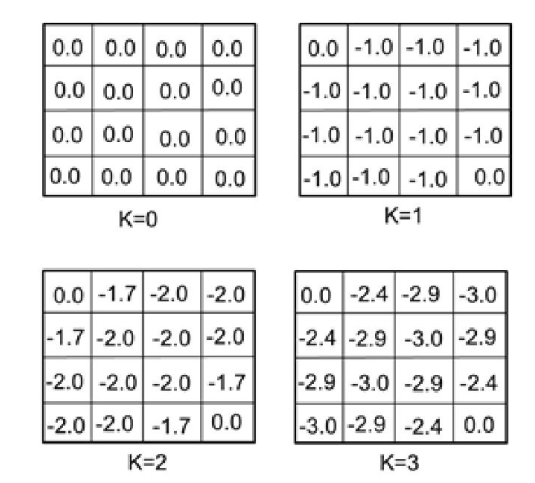状态1，第二次迭代的值函数的值计算过程如下：
v 2 ( 1 ) = 0.25 ( − 1 − 1 ) + 0.25 ( − 1 − 1 ) + 0.25 ( − 1 − 1 ) + 0.25 ( − 1 − 0 ) = − 1.75 ≈ − 1.7 \begin{aligned} v_2(1) &= 0.25(-1-1)+ 0.25(-1-1)+ 0.25(-1-1)+ 0.25(-1-0) \\ &= -1.75\approx-1.7 \end{aligned}
状态1，第三次迭代的值函数的值计算过程如下：
v 3 ( 1 ) = 0.25 ( − 1 − 1.7 ) + 0.25 ( − 1 − 2 ) + 0.25 ( − 1 − 2 ) + 0.25 ( − 1 − 0 ) = − 2.425 ≈ − 2.4 \begin{aligned} v_3(1) &= 0.25(-1-1.7)+ 0.25(-1-2)+ 0.25(-1-2)+ 0.25(-1-0) \\ &= -2.425\approx-2.4 \end{aligned}

## 3. 策略优化算法

现在我们来解决如何改善策略的问题。很自然的解决方案是采用贪心的策略来解决这个问题，也就是在每个状态都采用行为-状态值函数最大的动作，如下图所示：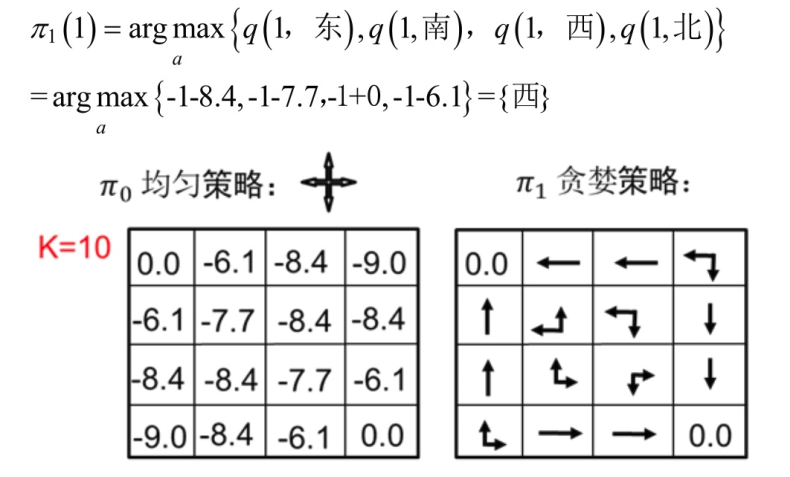## 4. 策略迭代算法和值函数迭代算法

所谓策略迭代是指先初始化策略、然后在每个策略下计算出收敛的状态值（采用策略评估算法），而后根据计算出的值使用策略优化算法修改策略，如此迭代，直到策略收敛，就作为最优策略，伪代码如下：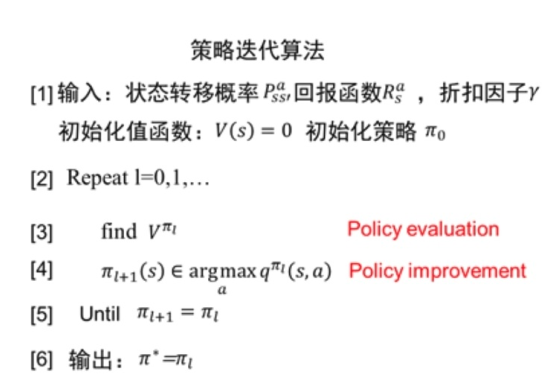值函数迭代算法也是先初始化策略，而后在每次更新状态的值的时候，直接采用当前状态下能够产生的最大值更新值（而不是向策略迭代算法中那样采用均值），直到值收敛后，采用一次策略优化获得最终的策略，伪代码如下：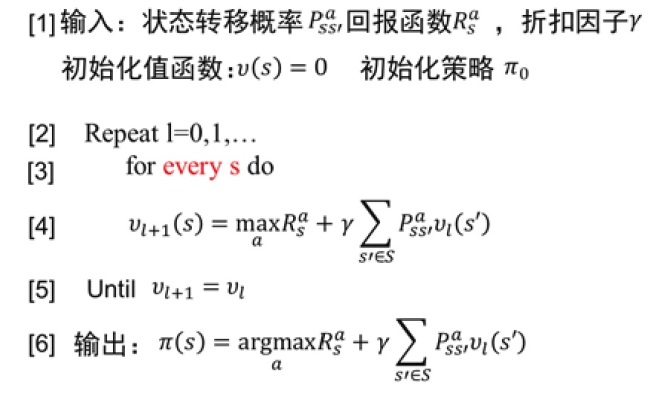## 5. 代码实现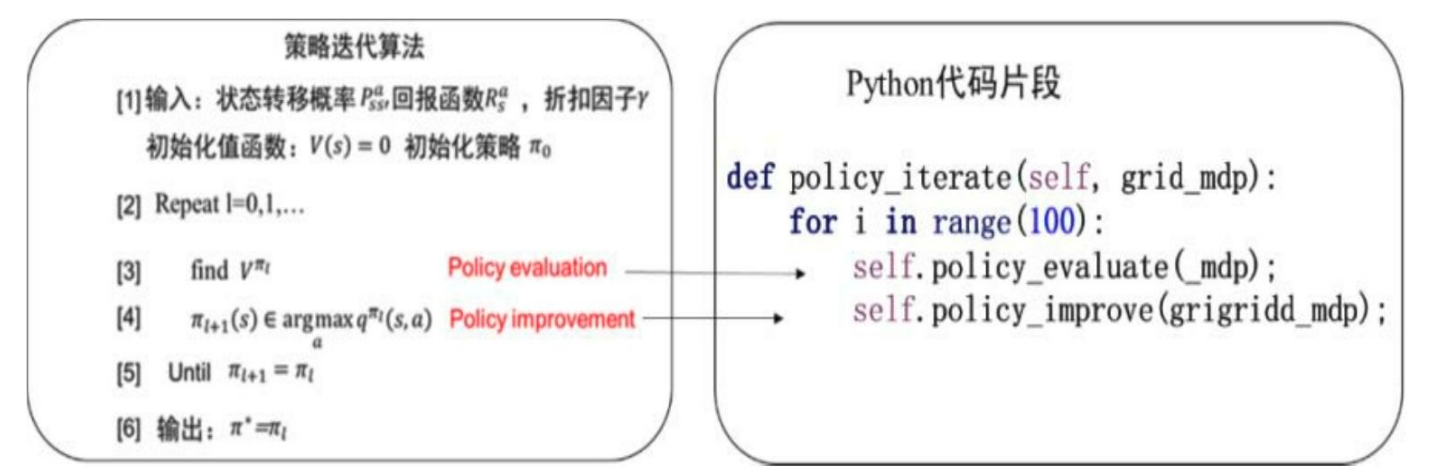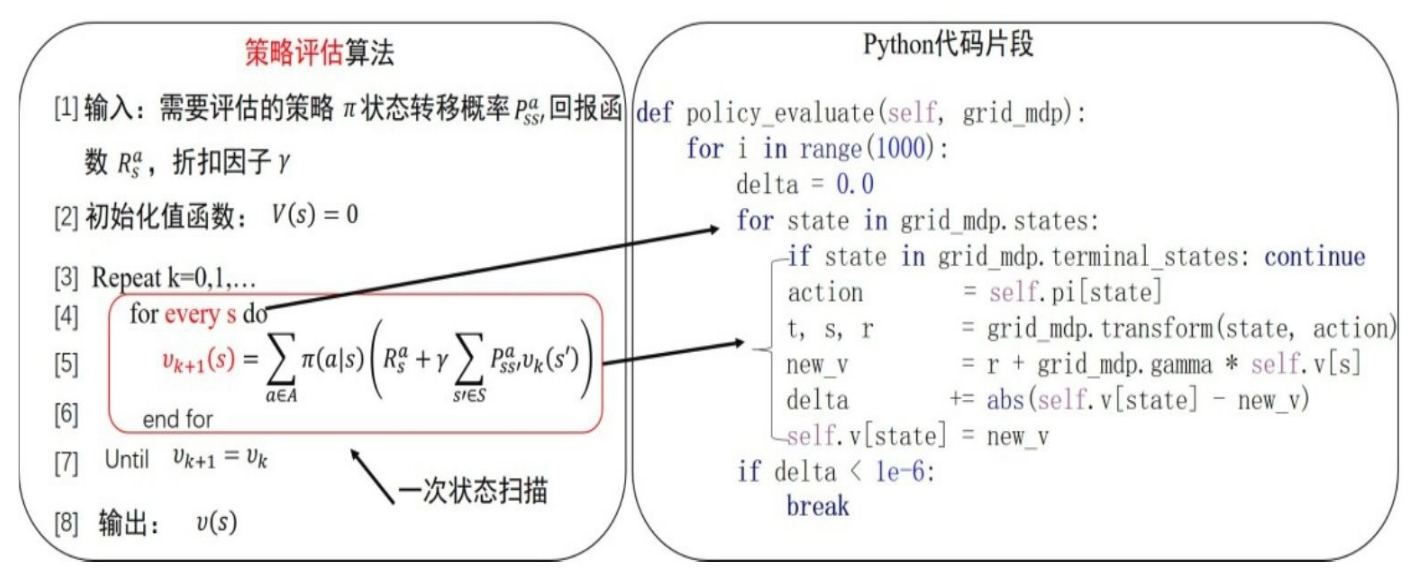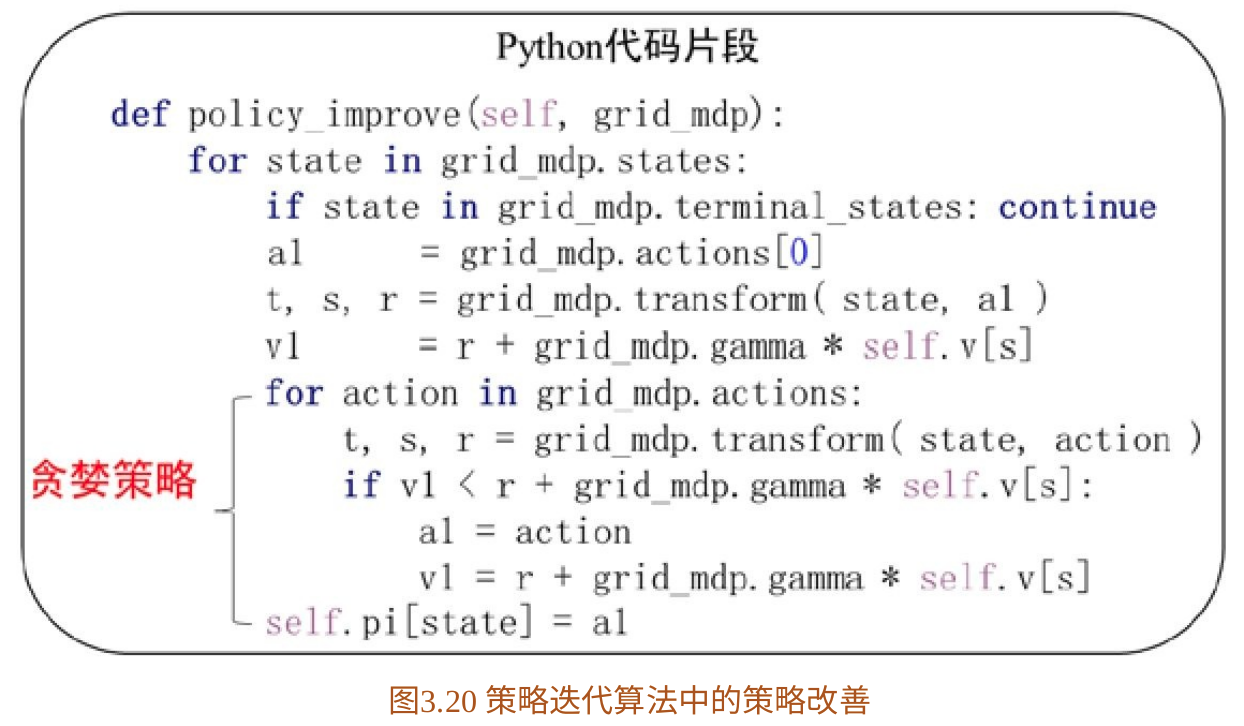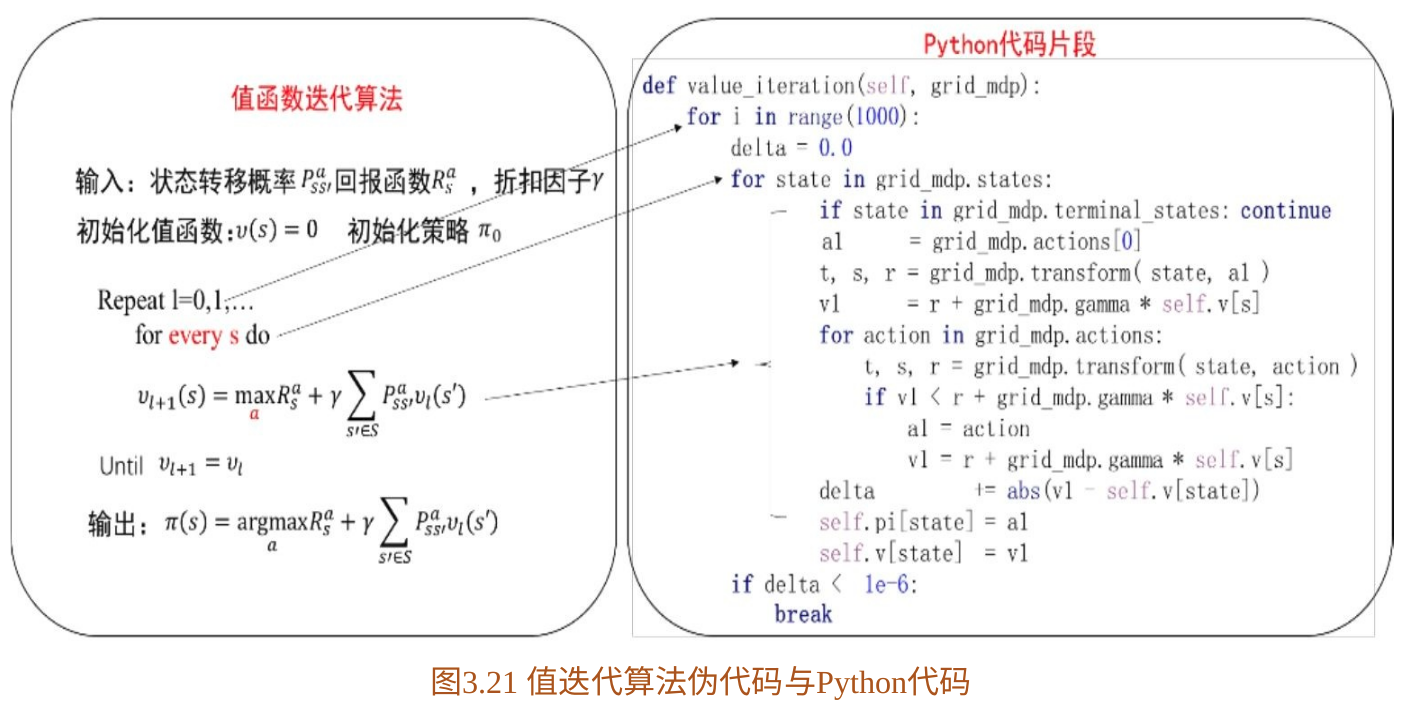## 6. 强化学习与最优控制

最优控制领域经过几十年的发展有许多的优秀成果，在基于模型的强化学习算法中可以利用这些优秀成果。基于模型的算法中，会先根据数据拟合出一个模型，根据已知的模型，最优控制领域中有很多好的方法可以计算出最优策略解，智能体再根据这些最优控制策略与环境交互，完成进一步优化。

结合最优控制的强化学习算法最典型的应用就是引导策略搜索算法。

展开全文强化学习 值迭代算法 策略评估算法
• 强化学习有两种常见迭代训练算法：策略迭代算法和值迭代算法。本文中主要讲述策略迭代算法。 先从一个简答的问题开始，下图为一个四方格子，每个位置的状态空间分别为{1, 2, 3, 4}, 其中 3 的位置是个陷阱， 4的...

强化学习有两种常见迭代训练算法：策略迭代算法和值迭代算法。本文中主要讲述策略迭代算法。

先从一个简答的问题开始，下图为一个四方格子，每个位置的状态空间分别为{1, 2, 3, 4}, 其中 3 的位置是个陷阱， 4的位置有个金币。有一个机器人从状态1的位置开始寻找金币。落入陷阱的回报为-1，找到金币的回报为1，在其他位置间移动回报为0，可选的动作空间为{上，下，左，右}， 通过这个简单的问题，来学习强化学习的学习原理。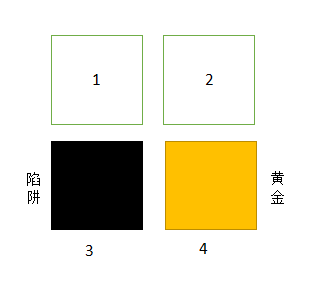强化学习的学习过程，个人理解就是通过不断的尝试，去更新每个状态的值函数(每个状态的值代表了当前状态的优劣，如果状态值很大，从其他状态选择一个动作，转移到该状态便是一个正确的选择)，然后通过更新后的值函数去动态的调整策略，在调整策略后，又去更新值函数，不断的迭代更新，最后训练完成一个满足要求的策略。在这个过程中，抽象出两个主要的过程，第一个叫策略评估，第二个叫策略改善。

针对上面给出的简单问题，先说明一些简单的概念:

#### 每个状态的值函数：

代表机器人处于该状态时的优劣值。

#### 针对问题的当前策略：

代表机器人处于某状态时，选择的下一步动作。对于选择的下一步动作，可以是确定式的，比如当机器人处于1位置的时候，确定的只选择往右走。也可以是概率式的，可以0.5的概率选择往右走， 0.5的概率选择往下走。当然确定式策略选择是概率式的策略选择的一种特例。下文中采用确定式策略进行描述

#### 策略评估：

策略评估就是通过某种方式，计算状态空间中每个状态的值函数。由于状态空间之间存在很多转移关系，要直接计算某个状态的值函数，是很困难的，一般采用
迭代方法。

#### 策略改善：

对策略的改善，即通过当前拥有的信息，对当前策略进行优化，修改当前策略。

###### ############################## 策略评估的过程

初始化的策略和值函数。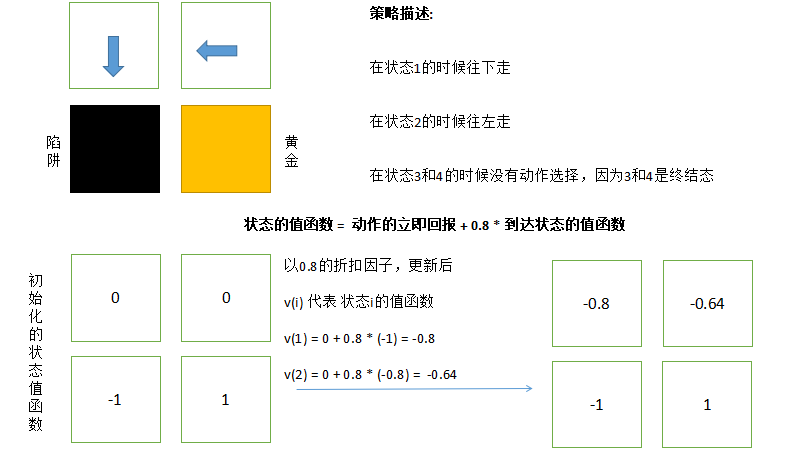对于这个简单的例子，通过一步计算便得到了稳定的值函数，但是对于大多数的问题，都需要通过多步的迭代，才能得到稳定的值函数。

###### ############################## 策略改善的过程

对于这个简单的例子，采用贪心的方式对策略进行改善，通过上一步策略评估过程计算出的稳定的值函数，让每个状态在选择下一步动作的时候，选择使动作收益最大的动作。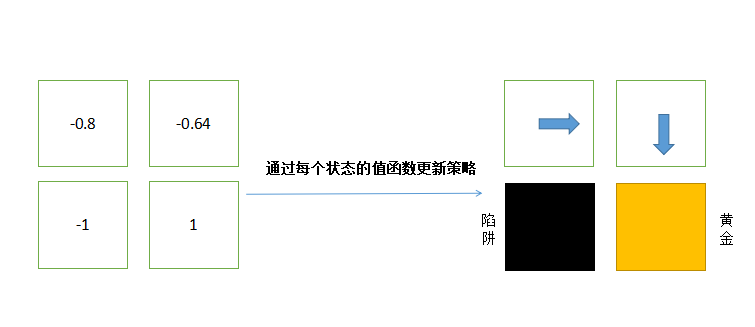#### 总结

强化学习策略迭代算法的过程就是不断的重复 策略评估 和 策略改善的过程，直到整个策略收敛（值函数和策略不再发生大的变化）

#### gym的样例代码展示

上述的简单示例，简单描述了强化学习的策略迭代算法的过程，下面将问题搞复杂一点，通过对该问题进行编程，加深对策略迭代算法的理解。新问题的空间状态如下图所示。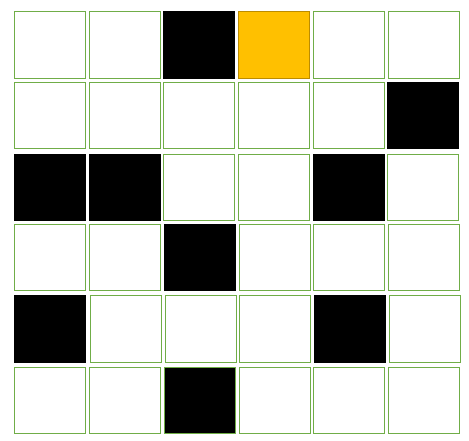该图的状态空间由下置上，从左到右，分别为1 – 36

import numpy as np
import random
from gym import spaces
import gym
from gym.envs.classic_control import rendering

#模拟环境类
class GridWorldEnv(gym.Env):
#相关的全局配置
'render.modes':['human', 'rgb_array'],
'video.frames_per_second': 2
}

def __init__(self):
self.states = [i for i in range(1, 37)] #初始化状态
self.terminate_states = [3, 7, 11, 15, 19, 20, 23, 30,  33, 34] #终结态
self.actions = ['up', 'down', 'left', 'right'] #动作空间

self.v_states = dict() #状态的值空间
for state in self.states:
self.v_states[state] = 0.0

for state in self.terminate_states: #先将所有陷阱和黄金的值函数初始化为-1.0
self.v_states[state] = -1.0

self.v_states = 1.0  #黄金的位置值函数初始化为 1

self.initStateAction() #初始化每个状态的可行动作空间
self.initStatePolicyAction() #随机初始化当前策略

self.gamma = 0.8 #计算值函数用的折扣因子
self.viewer = None #视图对象
self.current_state = None #当前状态
return

def translateStateToRowCol(self, state):
"""
将状态转化为行列坐标返回
"""
row = (state - 1) // 6
col = (state - 1) %  6
return row, col

def translateRowColToState(self, row, col):
"""
将行列坐标转化为状态值
"""
return row * 6 + col + 1

def actionRowCol(self, row, col, action):
"""
对行列坐标执行动作action并返回坐标
"""
if action == "up":
row = row - 1
if action == "down":
row = row + 1
if action == "left":
col = col - 1
if action == "right":
col = col + 1
return row, col

def canUp(self, row, col):
row = row - 1
return 0 <= row <= 5

def canDown(self, row, col):
row = row + 1
return 0 <= row <= 5

def canLeft(self, row, col):
col = col - 1
return 0 <= col <= 5

def canRight(self, row, col):
col = col + 1
return 0 <= col <= 5

def initStateAction(self):
"""
初始化每个状态可行动作空间
"""
self.states_actions = dict()
for state in self.states:
self.states_actions[state] = []
if state in self.terminate_states:
continue
row, col = self.translateStateToRowCol(state)
if self.canUp(row, col):
self.states_actions[state].append("up")
if self.canDown(row, col):
self.states_actions[state].append("down")
if self.canLeft(row, col):
self.states_actions[state].append('left')
if self.canRight(row, col):
self.states_actions[state].append('right')
return

def initStatePolicyAction(self):
"""
初始化每个状态的当前策略动作
"""
self.states_policy_action = dict()
for state in self.states:
if state in self.terminate_states:
self.states_policy_action[state] = None
else:
self.states_policy_action[state] = random.sample(self.states_actions[state], 1)
return

def seed(self, seed = None):
random.seed(seed)
return [seed]

def reset(self):
"""
重置原始状态
"""
self.current_state = random.sample(self.states, 1)

def step(self, action):
"""
动作迭代函数
"""
cur_state = self.current_state
if cur_state in self.terminate_states:
return cur_state, 0, True, {}
row, col = self.translateStateToRowCol(cur_state)
n_row, n_col = self.actionRowCol(row, col, action)
next_state = self.translateRowColToState(n_row, n_col)
self.current_state = next_state
if next_state in self.terminate_states:
return next_state, 0, True, {}
else:
return next_state, 0, False, {}

def policy_evaluate(self):
"""
策略评估过程
"""
error = 0.000001 #误差率
for _ in range(1000):
max_error = 0.0 #初始化最大误差
for state in self.states:
if state in self.terminate_states:
continue
action = self.states_policy_action[state]
self.current_state = state
next_state, reward, isTerminate, info = self.step(action)
old_value = self.v_states[state]
self.v_states[state] = reward + self.gamma * self.v_states[next_state]
abs_error = abs(self.v_states[state] - old_value)
max_error = abs_error if abs_error > max_error else max_error #更新最大值
if max_error < error:
break

def policy_improve(self):
"""
根据策略评估的结果，进行策略更新,并返回每个状态的当前策略是否发生了变化
"""
changed = False
for state in self.states:
if state in self.terminate_states:
continue
max_value_action = self.states_actions[state] #当前最大值行为
max_value = -1000000000000.0 #当前最大回报
for action in self.states_actions[state]:
self.current_state = state
next_state, reward, isTerminate, info = self.step(action)
q_reward = reward + self.gamma * self.v_states[next_state]
if q_reward > max_value:
max_value_action = action
max_value = q_reward
if self.states_policy_action[state] != max_value_action:
changed = True
self.states_policy_action[state] = max_value_action
return changed

def createGrids(self):
"""
创建网格
"""
start_x = 40
start_y = 40
line_length = 40
for state in self.states:
row, col = self.translateStateToRowCol(state)
x = start_x + col * line_length
y = start_y + row * line_length
line = rendering.Line((x, y), (x + line_length, y))
line.set_color(0, 0, 0)
line = rendering.Line((x, y), (x, y  + line_length))
line.set_color(0, 0, 0)
line = rendering.Line((x + line_length, y), (x + line_length, y  + line_length))
line.set_color(0, 0, 0)
line = rendering.Line((x, y + line_length), (x + line_length, y  + line_length))
line.set_color(0, 0, 0)

def createTraps(self):
"""
创建陷阱,将黄金的位置也先绘制成陷阱，后面覆盖画成黄金
"""
start_x = 40
start_y = 40
line_length = 40
for state in self.terminate_states:
row, col = self.translateStateToRowCol(state)
trap = rendering.make_circle(20)
trans = rendering.Transform()
trap.set_color(0, 0, 0)
trans.set_translation(start_x + line_length * col + 20, start_y + line_length * row + 20)

def createGold(self):
"""
创建黄金
"""
start_x = 40
start_y = 40
line_length = 40
state = 34
row, col = self.translateStateToRowCol(state)
gold = rendering.make_circle(20)
trans = rendering.Transform()
gold.set_color(1, 0.9, 0)
trans.set_translation(start_x + line_length * col + 20, start_y + line_length * row + 20)

def createRobot(self):
"""
创建机器人
"""
start_x = 40
start_y = 40
line_length = 40
row, col = self.translateStateToRowCol(self.current_state)
robot = rendering.make_circle(15)
trans = rendering.Transform()
robot.set_color(1, 0, 1)
trans.set_translation(start_x + line_length * col + 20, start_y + line_length * row + 20)

def render(self, mode="human", close=False):
"""
渲染整个场景
"""
#关闭视图
if close:
if self.viewer is not None:
self.viewer.close()
self.viewer = None

#视图的大小
screen_width = 320
screen_height = 320

if self.viewer is None:
self.viewer = rendering.Viewer(screen_width, screen_height)

#创建网格
self.createGrids()
#创建陷阱
self.createTraps()
#创建黄金
self.createGold()
#创建机器人
self.createRobot()
return self.viewer.render(return_rgb_array= mode == 'rgb_array')
##### 注册环境模拟类到gym
from gym.envs.registration import register
try:
register(id = "GridWorld-v3", entry_point=GridWorldEnv, max_episode_steps = 200, reward_threshold=100.0)
except:
pass
##### 进行策略迭代算法的过程和模拟动画的代码
from time import sleep
env = gym.make('GridWorld-v3')
env.reset()

#策略评估和策略改善
not_changed_count = 0
for _ in range(10000):
env.env.policy_evaluate()
changed = env.env.policy_improve()
if changed:
not_changed_count = 0
else:
not_changed_count += 1
if not_changed_count == 10: #超过10次策略没有再更新，说明策略已经稳定了
break

#观察env到底是个什么东西的打印信息。
print(isinstance(env, GridWorldEnv))
print(type(env))
print(env.__dict__)
print(isinstance(env.env, GridWorldEnv))

env.reset()

for _ in range(1000):
env.render()
if env.env.states_policy_action[env.env.current_state] is not None:
observation,reward,done,info = env.step(env.env.states_policy_action[env.env.current_state])
else:
done = True
print(_)
if done:
sleep(0.5)
env.render()
env.reset()
print("reset")
sleep(0.5)
env.close()
##### 动画效果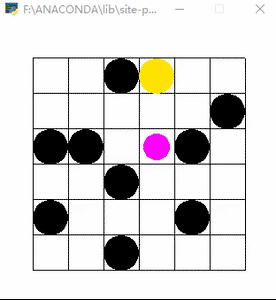展开全文• 用最简单的python语法实现小网格环境下的迭代策略评估（Iterative Policy Evaluation in Small Gridworld） 一 、小网格环境下的迭代策略简介 废话不多说，能看到这篇博客的应该都对这张两图再熟悉不过。 在...

# 一 、小网格环境下的迭代策略简介

哈哈，能看到这篇博客的应该都对这张两图再熟悉不过。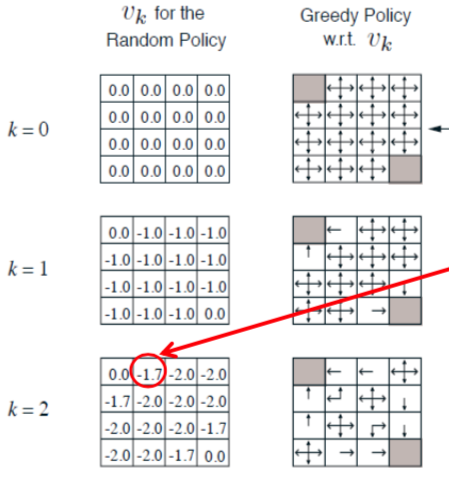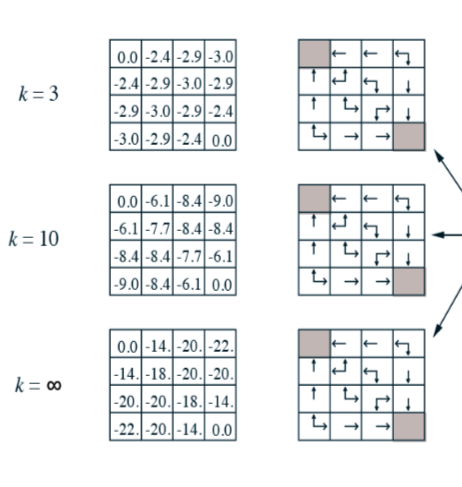在阅读此篇博客之前，希望你已经对马尔可夫决策以及贝尔曼方程有初步的了解。

在此基础上，通过这篇博客，你可以学会以下几点。

• 那个-1.7究竟是怎么算的？（其实并不是-1.7）
• k取任意值时每个方格里面每个值是怎么算的？
• 如何用python计算任意次迭代次数（k取任意值）后这个网格中任意方格中的值？
-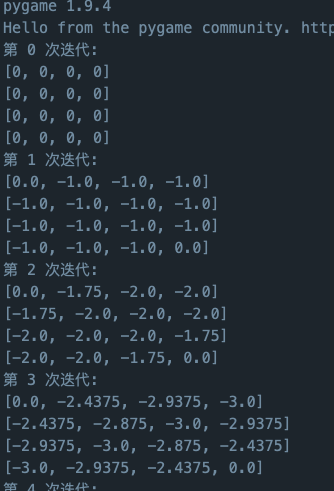• 如何用python实现对于任意大小的网格，完成上步操作？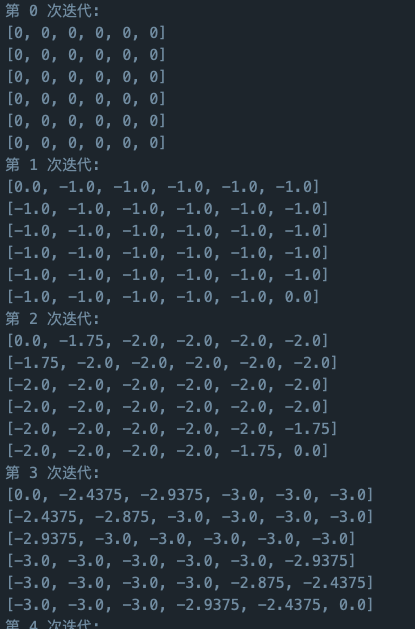• 如何用python实现此游戏的的可视化？当然啦，网格的大小你可以自己调，同时除了马尔可夫决策外，我还准备了随机决策。通过可视化，你可以看到小方块在两种策略下具体是怎么移动的。
•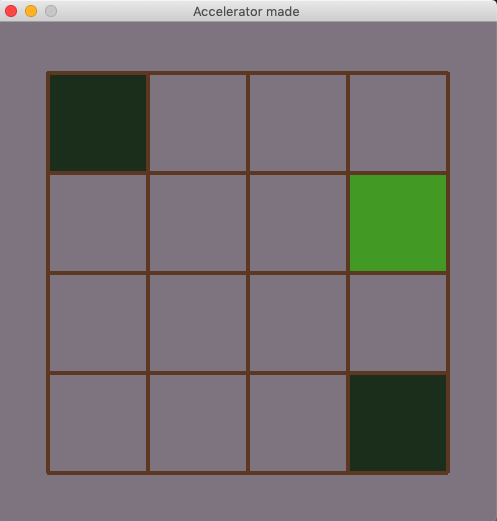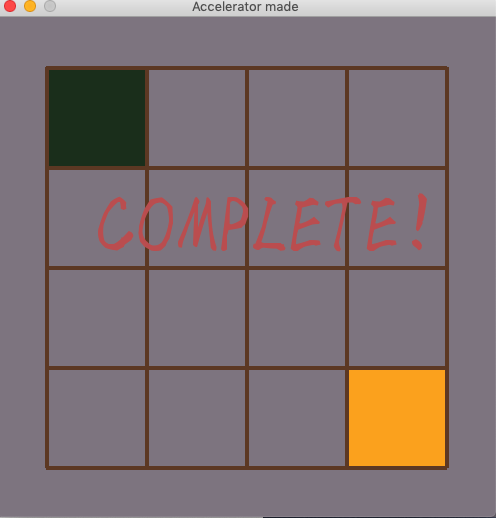•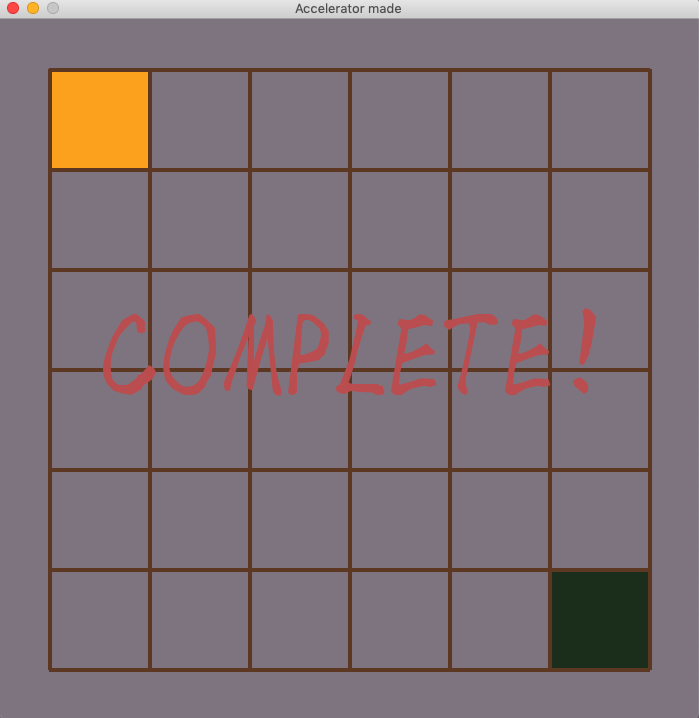# 二、问题重述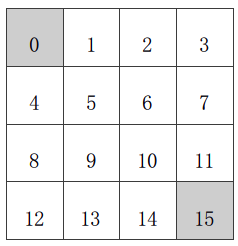已知（如上图）：

• 状态空间 S：S_1 - S_14为非终止状态；S_0，S_15 终止状态，图中灰色方格所示两个位置；
• 行为空间 A：{n, e, s, w} 对于任何非终止状态可以有向北、东、南、西移动四个行为；
• 转移概率 P：任何试图离开方格世界的动作其位置将不会发生改变，其余条件下将100%地转移到动作指向的位置；
• 即时奖励 R：任何在非终止状态间的转移得到的即时奖励均为-1，进入终止状态即时奖励为0；
• 衰减系数 γ：1；
• 当前策略π：个体采用随机行动策略，在任何一个非终止状态下有均等的几率往任意可能的方向移动，即π(n|•) = π(e|•) = π(s|•) = π(w|•) = 1/4。
• 问题：评估在这个方格世界里给定的策略。
• 该问题等同于：求解该方格世界在给定策略下的（状态）价值函数，也就是求解在给定策略下，该方格世界里每一个状态的价值。

# 三、如何计算每个方块的状态的价值？

给出通俗易懂的解释：
这个格子在k=n时的价值等于（它到周围格子的策略价值总和再加上及时奖励的价值总和）/4。特别的，它如果撞墙了，则回到自身，同样也有-1的及时奖励，而回到自身所产生的策略价值就是k=n-1时的这个各自的价值。
给出通俗易懂的公式：
用Pn(i,j)表示坐标为(i,j)的格子在k=n时的策略价值，则

P n ( i , j ) = P n − 1 ( i − 1 , j ) + P n − 1 ( i + 1 , j ) + P n − 1 ( i , j − 1 ) + P n − 1 ( i , j + 1 ) − 4 4 P_{n}(i, j)=\frac{P_{n-1}(i-1, j)+P_{n-1}(i+1, j)+P_{n-1}(i, j-1)+P_{n-1}(i, j+1)-4}{4}

以下图红圈内的格子为例：
P=（0-1-1-1-4）/4=-1.75，所以这里的-1.7的确切值应该是-1.75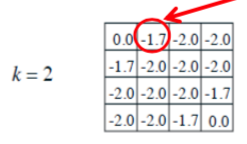以下图红圈内的格子为例：
P=（0-1.75-2-2-4）/4=-2.4375，所以这里的-2.4的确切值应该是-2.4375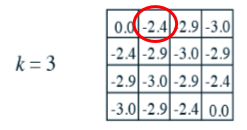# 四、python求解任意棋盘大小任意k值任意网格的价值

定义一个评分函数，传入参数是棋盘的大小，题目中棋盘大小为4*4，所以chess_number为4。之后定义两个二维数组，用于存放每个格子的价值以及更新后的价值，均赋0备用。

def chess_score(chess_number):
global score
global newscore
for i in range(chess_number):
score.append([])
for j in range(chess_number):
score[i].append(0)
for i in range(chess_number):
newscore.append([])
for j in range(chess_number):
newscore[i].append(0)


设置迭代次数，因为有限过程的markov过程是收敛的，依据经验本约束条件下大约150次左右价值趋于稳定,所以这里我设置iteration为150.
之后遍历棋盘判断所有点的价值。
除了终点外，我将任意大小的棋盘分成九个区域，分别是右上角，中上，左上角，左中，右中，左下角，中下，右下角，以及通常。之后分类套公式，再将更新的价值存入newscore中。那么为什么不直接存入score中呢，因为如果立刻存入score，在一次迭代没有结束时，这个点的价值会影响后面未更新点的价值，导致结果出错。

	for iteration in range(150):
print("第",iteration,"次迭代:")
for i in range(chess_number):
print(score[i])
for i in range(chess_number):
for j in range(chess_number):
if [i,j] in endpos1:#吸收态坐标
score[i][j]=0.0
else:
if(i==0 and j==0):#左上
newscore[i][j]=round((score[i][j]+score[i+1][j]+score[i][j+1]+score[i][j]-4)/4.0,5)
elif (i==0 and j!=0 and j!=chess_number-1):#中上
newscore[i][j]=round((score[i][j]+score[i+1][j]+score[i][j+1]+score[i][j-1]-4)/4.0,5)
elif (i==0 and j==chess_number-1 ):#右上
newscore[i][j]=round((score[i][j]+score[i+1][j]+score[i][j]+score[i][j-1]-4)/4.0,5)
elif (i!=0 and i!=chess_number-1 and j==0 ):#左中
newscore[i][j]=round((score[i-1][j]+score[i+1][j]+score[i][j+1]+score[i][j]-4)/4.0,5)
elif (i!=0 and i!=chess_number-1 and j!=0 and j!=chess_number-1):#normal
newscore[i][j]=round((score[i-1][j]+score[i+1][j]+score[i][j+1]+score[i][j-1]-4)/4.0,5)
elif (i!=0 and i!=chess_number-1 and j==chess_number-1):#右中
newscore[i][j]=round((score[i-1][j]+score[i+1][j]+score[i][j]+score[i][j-1]-4)/4.0,5)
elif (i==chess_number-1 and j==0):#左下
newscore[i][j]=round((score[i-1][j]+score[i][j]+score[i][j+1]+score[i][j]-4)/4.0,5)
elif (i==chess_number-1 and j!=0 and j!=chess_number-1):#中下
newscore[i][j]=round((score[i-1][j]+score[i][j]+score[i][j+1]+score[i][j-1]-4)/4.0,5)
elif (i==chess_number-1 and j==chess_number-1):#右下
newscore[i][j]=round((score[i-1][j]+score[i][j]+score[i][j]+score[i][j-1]-4)/4.0,5)


更新score的值，没啥好说的。

	for i in range(chess_number):
for j in range(chess_number):
if newscore[i][j]!=0:
score[i][j]=newscore[i][j]
newscore[i][j]=0


# 五、python实现该游戏的可视化

首先说说可以实现哪些内容吧。

• 可以自己设置网格的大小。
• 实现随机决策下随机格子走到终点的动画
• 实现马尔可夫决策下随机格子走到终点的动画

### 准备工作

这里我是用pygame做的，所以要导入pygame哦，没装的同学要记得先装一下，网上教程很多，这里就不再赘述。

import pygame
import sys
import math
import random

# pygame 初始化
pygame.init()
# 设置棋盘大小
chess_number=6
# 设置是否使用马尔可夫决策
usemarkov=1#等于1使用，等于0不使用

BG=(144,136,145)#背景色
LINECOLOR=(112,73,46)#网格色
STEPCOLOR=(79,167,47)#随机格子的颜色
STARTCOLOR=(119,207,87)#你想实现格子轨迹的时候用介个颜色好看
ENDCOLOR=(34,60,36)#吸收态格子的颜色
WINCOLOR=(253,176,36)#随机格子落入吸收态的颜色
WALLCOLOR=(112,73,46)#墙壁的颜色，本来还想实现障碍物的，介个就交给你们啦
TEXTCOLOR=(200,98,96)#文本的颜色
#设置起始位置（左上角）
START_POS=(50,50)
START_POSX=50
START_POSY=50
CELL_LENGTH=100#每个格子的像素大小
LINE_WIDTH=4#线的宽度
# 设置背景框大小
size = width, height = 2*START_POSX+chess_number*CELL_LENGTH,2*START_POSY+chess_number*CELL_LENGTH

# 设置帧率，返回clock 类
clock = pygame.time.Clock()

screen = pygame.display.set_mode(size)
# 设置起始位置
start_posx=random.randint(1,chess_number)
start_posy=random.randint(1,chess_number)
#设置位置
pos=[start_posx,start_posy]
endpos=[[1,1],[chess_number,chess_number]]#可视化用
endpos1=[[0,0],[chess_number-1,chess_number-1]]#价值计算用
#设置分数
score=[]
newscore=[]
#初始化步数
step=0



### 画图函数

画网格线啦，画各种格子啦，显示完成后的文字“COMPLETE！”啦
在一开始的时候step为0，画起点，之后通过判断usemarkov的值来确定决策种类。更新方块儿位置。

def draw():
global pos
global step
for i in range(chess_number+1):
pygame.draw.line(screen, LINECOLOR, (START_POSX,START_POSY+i*CELL_LENGTH), (START_POSX+chess_number*CELL_LENGTH,START_POSY+i*CELL_LENGTH), LINE_WIDTH)#横线
pygame.draw.line(screen, LINECOLOR, (START_POSX+i*CELL_LENGTH,START_POSY),(START_POSX+i*CELL_LENGTH,START_POSY+chess_number*CELL_LENGTH), LINE_WIDTH)#竖线#
drawcell(chess_number, chess_number, ENDCOLOR) #终点
drawcell(1, 1, ENDCOLOR)#终点
if step==0:
drawcell(pos,pos, STEPCOLOR)#起点
#drawcell(start_posx, start_posy, STARTCOLOR)#起点
else:
if (pos!=endpos and pos!=endpos):
print(pos)
if usemarkov==0:
pos=nologic(pos)#随机决策
else:
pos=markov(pos)#马尔可夫决策
else:
drawcell(pos, pos, WINCOLOR)#终点
my_font=pygame.font.Font('mufont.ttf',20*chess_number)
text=my_font.render("COMPLETE！",True,TEXTCOLOR)
screen.blit(text,(100,height/2-100))
step+=1



### 具体画格子的方法

在pygame中很简单，就是画一个矩形就行

def drawcell(i,j,cellkind):#行，列，方块种类
pygame.draw.rect(screen,cellkind,[START_POSX+CELL_LENGTH*(j-1)+(LINE_WIDTH-1),START_POSY+CELL_LENGTH*(i-1)+(LINE_WIDTH-1),CELL_LENGTH-LINE_WIDTH,CELL_LENGTH-LINE_WIDTH],0)#终点.


### 实现随机决策的方法

很简单，思想前面已经说过了，将棋盘分成九个区域，对于每个区域的格子判断可能的行走方向，再随机取一个就OK。

def nologic(pos):
i=pos
j=pos
#drawcell(i,j, STARTCOLOR)#下
if   (i==1 and j==1):#左上
print("左上")
godnum=random.randint(1,2)
if godnum==1:
drawcell(i,j+1, STEPCOLOR)#下
pos=[i,j+1]
elif godnum==2:
drawcell(i,j+1, STEPCOLOR)#右
pos=[i,j+1]
elif (i==1 and j!=1 and j!=chess_number):#中上
print("中上")
godnum=random.randint(1,3)
if godnum==1:
drawcell(i,j+1, STEPCOLOR)#下
pos=[i,j+1]
elif godnum==2:
drawcell(i,j+1, STEPCOLOR)#右
pos=[i,j+1]
elif godnum==3:
drawcell(i,j-1, STEPCOLOR)#左
pos=[i,j-1]
elif (i==1 and j==chess_number ):#右上
print("右上")
godnum=random.randint(1,2)
if godnum==1:
drawcell(i+1,j, STEPCOLOR)#下
pos=[i+1,j]
elif godnum==2:
drawcell(i,j-1, STEPCOLOR)#左
pos=[i,j-1]
elif (i!=1 and i!=chess_number and j==1 ):#左中
print("左中")
godnum=random.randint(1,3)
if godnum==1:
drawcell(i+1,j, STEPCOLOR)#下
pos=[i+1,j]
elif godnum==2:
drawcell(i,j+1, STEPCOLOR)#右
pos=[i,j+1]
elif godnum==3:
drawcell(i-1,j, STEPCOLOR)#上
pos=[i-1,j]
elif (i!=1 and i!=chess_number and j!=1 and j!=chess_number):#normal
print("normal")
godnum=random.randint(1,4)
if godnum==1:
drawcell(i+1,j, STEPCOLOR)#下
pos=[i+1,j]
elif godnum==2:
drawcell(i,j+1, STEPCOLOR)#右
pos=[i,j+1]
elif godnum==3:
drawcell(i,j-1, STEPCOLOR)#左
pos=[i,j-1]
elif godnum==4:
drawcell(i-1,j, STEPCOLOR)#上
pos=[i-1,j]
elif (i!=1 and i!=chess_number and j==chess_number):#右中
print("右中")
godnum=random.randint(1,3)
if godnum==1:
drawcell(i+1,j, STEPCOLOR)#下
pos=[i+1,j]
elif godnum==2:
drawcell(i,j-1, STEPCOLOR)#左
pos=[i,j-1]
elif godnum==3:
drawcell(i-1,j, STEPCOLOR)#上
pos=[i-1,j]
elif (i==chess_number and j==1):#左下
print("左下")
godnum=random.randint(1,2)
if godnum==1:
drawcell(i,j+1, STEPCOLOR)#右
pos=[i,j+1]
print("往右边走")
elif godnum==2:
drawcell(i-1,j, STEPCOLOR)#上
pos=[i-1,j]
print("往上边走")
elif (i==chess_number and j!=1 and j!=chess_number):#中下
print("中下")
godnum=random.randint(1,3)
if godnum==1:
drawcell(i,j+1, STEPCOLOR)#右
pos=[i,j+1]
print("往右边走")
elif godnum==2:
drawcell(i,j-1, STEPCOLOR)#左
pos=[i,j-1]
print("往左边走")
elif godnum==3:
drawcell(i-1,j, STEPCOLOR)#上
pos=[i-1,j]
print("往上边走")
elif (i==chess_number and j==chess_number):#右下
print("右下")
godnum=random.randint(1,2)
if godnum==1:
drawcell(i,j-1, STEPCOLOR)#左
pos=[i,j-1]
print("往左边走")
elif godnum==2:
drawcell(i-1,j, STEPCOLOR)#上
pos=[i-1,j]
print("往上边走")
return pos


### 实现马尔可夫决策的方法

有了上面价值表作为基础，实现马尔可夫决策就更简单啦，只需要判断方块儿上下左右的价值是否比自己价值大，然后往价值大的地方走就OK啦。

def markov(pos):
i=pos-1
j=pos-1
print(pos)
print(score[i][j-1],score[i][j])
if score[i-1][j]>score[i][j] and i-1>=0:
drawcell(i,j+1, STEPCOLOR)
print("往上走")
pos=[i,j+1]
return pos
if i+1<chess_number and score[i+1][j]>score[i][j]:
drawcell(i+2,j+1, STEPCOLOR)
print("往下走")
pos=[i+2,j+1]
return pos
if score[i][j-1]>score[i][j]:
drawcell(i+1,j, STEPCOLOR)
print("往左走")
pos=[i+1,j]
return pos
if j+1<chess_number and score[i][j+1]>score[i][j]:
drawcell(i+1,j+2, STEPCOLOR)
print("往右走")
pos=[i+1,j+2]
return pos


好啦，到此为止，小网格环境下的迭代策略评估的价值计算以及python可视化的实现就算全部完成啦。如果有什么疏漏之处或者好的建议，也欢迎指正哦。

# 配套源码已经上传CSDN啦，直接下载就可以，谢谢大家支持。

展开全文马尔可夫决策 python
• 提示：转载请注明出处，若文章无意侵犯到您...在之前的学习中我们学习了有限MDP问题的基于模型的DP解法，也就是策略迭代法，但是很多东西不与实践代码相结合，总会觉得没有成就感，那么我们就将之前介绍的算法通过举...机器学习 强化学习
• 这一发现表明，使用低差异网格策略迭代算法可能会成功打破“平均情况”设置中的维数诅咒，因为在多变量问题中，这些方法的收敛速度超过了基于随机网格的方法的收敛速度，其他确定性选择的网格，因此在 MDP 问题的...
• #创建网格世界，一共11条直线 self.line1=rendering.Line((100,300),(500,300)) self.line2=rendering.Line((100,200),(500,200)) self.line3=rendering.Line((100,100),(100,300)) self.line4=rendering.Line...人工智能 强化学习 机器学习 动态规划
• 本篇用代码演示《强化学习》第三讲中的示例——方格世界，即用动态规划算法通过迭代计算来评估4*4方格世界中的一个随机策略。具体问题是这样： 已知（如上图）： 状态空间 S：S_{1} - S_{14}为非终止状态；S_{0} ，...
• 本篇用代码演示David Silver《强化学习RL》第三讲 动态规划寻找最优策略中的示例——方格世界，即用动态规划算法通过迭代计算来评估4*4方格世界中的一个随机策略。具体问题是这样： 已知（如上图）： 状态空间 S...
• 动态规划就是基于模型的强化学习方法，可分为策略迭代（policy iteration）和价值迭代（value iteration）两种，下面详细介绍。 \\[30pt] 1. 策略迭代 策略迭代又分为两个部分，即策略评估和策略改进。机器学习
• 在解决了单步决策问题以后，我们可以将多步问题分解为多个单步问题进行处理。使用ε-greedy等策略的基础在于对智能体当前的状态有良好的估计。如何对不同的状态均形成良好的估计呢，Q学习应运而生。 本文将主要介绍...matlab 强化学习 机器学习
• 中已经详细描述了策略迭代算法，其实值迭代算法和策略迭代算法的基本思想是一致的，其最大的区别在于，策略迭代算法在进行策略改善的时候，使用的每个状态的值函数，是稳定的，在进行策略评估的时候，计算得到了当前...
• 由于本章内容繁杂，篇幅较长，故分成了四部分来讲解，各部分主要内容分别为：交错网格、同位网格、边界条件、SIMPLE家族算法。 这里是第二部分，主要讲解交错网格的缺陷，以及如何不用交错网格，而直接在原来的同位...
• 本文主要对动态规划算法做以介绍与总结，并利用该算法实现对网格问题、杰克租车问题以及赌徒问题的仿真，其中尤其对于赌徒问题笔者进行了几个拓展思考，但毕竟水平有限，不尽人意之处还望各位海涵斧正。文章的开头...matlab python
• 文章目录一、超参二、网格搜索 GridSearchCV三、随机搜索 RandomizedSearchCV四、自动超参搜索：遗传算法（GA） 一、超参 学习器模型中一般有两类参数，一类是可以从数据中学习估计得到，我们称为参数（Parameter）...
• %利用delauny算法，生成三角形网格 triangulation_count = triangulation_count + 1; pmid = ( p(t(:,1),:) + p(t(:,2),:) + p(t(:,3),:) ) / 3; %计算三角形的重心。 t = t( feval_r( fd, pmid, varargin{:} ) , :...
• 递归是利用系统的堆栈保存函数当中的局部变量来解决问题的。递归就是在栈处理栈上一堆的指针指向内存中的对象，这些对象一直不被释放，直到递归执行到最后一次后，才释放空间.关于程序算法艺术与实践更多讨论与交流...
• 迭代算法(iteration) 迭代是重复反馈过程的活动，其目的通常是为了逼近所需目标或结果（一个判断条件）。每一次对过程的重复称为一次“迭代”，而每一次迭代得到的结果会作为下一次迭代的初始值。 递归算法...
• 网格计算, 云计算, 集群计算, 分布式计算, 超级计算   整体来说都有将任务分割、运算、组合，只是协同和处理的重点不同； 超级计算强调的是高并行计算能力，应用设备多是超级计算机如天河一号，是...
• 1. 软件工程和计算机有什么区别？ 基础课程重复度较高。 计算机偏学术研究，软件工程偏工程实践。一般来说计算机的学习偏重学习计算机的...2. 算法的基本特征和复杂度 （1）基本特征   输入、输出、有穷性、确
• 论文解读《通过迭代特征表示计算预测物种特异性酵母DNA复制起源》python
• 虽然较好地辅助了电子产品的设计，但是该方法仍存在许多缺陷，如需要进行复杂的网格剖分、迭代计算计算过程复杂、计算周期长。神经网络具有万能逼近和高效推理能力，这使得神经网络在求解微分方程时具有潜在的优势...AI
• 研究了基于自适应网格技术的结构拓扑优化．采用有限元离散设计域，单元节点密度作为...算例结果表明提出的拓扑优化策略可以减少结构分析和优化求解的计算量，在同等结构分析和优化求解计算量下能够得到更好的拓扑结果．
• 经研究，其和挖洞策略有关系，修改需要动源代码，十分费工夫。foam-extend-4.1版本有比较好的重叠网格功能，挖洞也很完善，能挖到边缘，是比较标准的，但extend比较大杂烩，也没有其他一些我需要的功能，所以只好...openfoam
• 本文简要介绍了网格简化的基础及意义，重点介绍了边坍缩（Edge Collapse）算法以及具体实现细节。
• 本文章收录在黑鲸智能系统知识库-黑鲸智能系统知识库成立于2021年，致力于建立一个完整的智能系统知识库体系。我们的工作：收集和整理...使用行为策略使Agent与环境互动，Agent将获得奖励，并能了解环境的一些情况,.强化学习 机器学习
• The grid-based technique is used for a multi-dimensional dataset. In this technique, we create a grid structure, and the comparison is performed on grids(also know as cells)....基于网格聚类 python 无监督学习 人工智能
• 改进思路 为了克服这些问题，论文提出了一种通用的Transformer骨干网，称为Swin Transformer，它构造了分层的特征映射，并且计算复杂度与图像大小成线性关系。 如图1(A)所示，Swin Transformer通过从小块(灰色轮廓)...大数据 编程语言 python 计算机视觉
• 我发现他的一系列文章都挺好，就是总缺点... 本文的算法来源于 Michael Garland在97年的文章 Surface simplification using quadric error metrics 算法介绍在计算机图形应用中，为了尽可能真实呈现虚拟物体，往往...